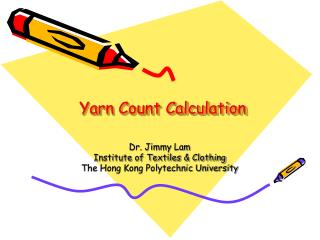DownloadDownload PresentationYarn Count Calculation

# Yarn Count Calculation

Télécharger la présentation## Yarn Count Calculation

- - - - - - - - - - - - - - - - - - - - - - - - - - - E N D - - - - - - - - - - - - - - - - - - - - - - - - - - -
##### Presentation Transcript

1. Yarn Count Calculation Dr. Jimmy Lam Institute of Textiles & Clothing The Hong Kong Polytechnic University

2. Outlines • What is yarn count? • Direct system and indirect system • Single yarn and ply yarn calculation • Metric system, Tex system and Cotton system conversion • Examples

3. Formula • Tex = mass (g)/ length (m) X 1000 • Conversion from Tex to other systems • Tex X Count =590.5 (Cotton System) • Tex X 9 = Denier (Filament System) • Tex X Metric Count =1000 (Knitwear system)

4. Yarn Count • Two Systems, namely Indirect System and Direct System • In sweater calculation, we normally converted both into Metric system (Nm)

5. Indirect System • Normally uses for cotton yarn and most of cotton blended yarns (acrylic cotton, ramie cotton yarn) • Symbol: NeC or ECC or ‘s • Definition: Number of unit length per unit mass (variable length, constant weight) • The higher the count number, the finer the yarn. • The lower the count number, the coarser the yarn.

6. Indirect System • Cotton Count (NeC) = Length (yd) x 840 /weight (pound) • Metric system (Nm)=Length (km) per weight (1 kg) • Conversion 1 Nm =NeC X 1.693

7. Indirect SystemExample • A skein of 26,880 yards weight 1 pound, calculate its English count and Metric equivalent. • Answer: • Cotton Count=26,880/840 =32NeC • Metric (Nm) =32 x 1.693 =54Nm

8. Direct System • Normally uses for continuous filament yarns such as viscose rayon, polyester, etc. • Definition: Number of mass per unit length (Variable weight and constant length) • The higher the count number, the coarser the yarn. • The lower the count number, the finer the yarn.

9. Direct System • Denier (Td) = weight (g) per length (m) x 9000 • Tex = weight (g) per length (m) x 1000 • Conversion to Metric System (Nm) • Nm=9000 /denier (Td) • Nm=1000/Tex (Tt) • Conversion between Tex and Denier • Tex=Denier/9

10. Direct SystemExample • A skein of 300 meters of filaments viscose rayon yarn weights 10 grams, calculate its denier and metric equivalent. • Answer: • Denier = 10 /300 x 9000 = 300Td • Metric (Nm) = 9000/300 =30Nm

11. More Example • Ex1: 20/2 NeC 55% acrylic, 45% cotton yarn • Metric System = 2/(20 x 1.693) =2/34Nm =17 Nm • Ex2: 11/2 NeC 55% ramie, 45% cotton yarn • Metric System= 2/(11 x.1693) • =2/18.6Nm

12. Resultant Count • Yarn produced by the combination of two or more single yarns is used in many textile structures in order to achieve effects which may not be readily obtained by single yarn. • In an indirect system, the resultant count is equal to the sum of the reciprocals of the component yarns. • 1/N = 1/N1 + 1/N2 where N is resultant count and N1 and N2 are component yarns

13. Resultant CountExample • A two fold yarn (ie yarn made by twisting two single yarn together) is found to have one 300 Td 100% viscose rayon yarn and one 20/1 NeC 100% cotton yarn. Calculate the equivalent single count in metric system. • Answer: • Metric (Nm) for rayon =9000/300=30Nm • Metric (Nm) for cotton= 20 x 1.693 =33.87Nm • Resultant Count 1/N = 1/30 + 1/33.87 • =1/15.9Nm

14. Yarn component calculation • A sweater is knitted with two different yarns. One end is 32/2 NeC 100% cotton yarn and the other end is of plated 84 Denier (Td) 85% nylon, 15% lycra yarn. Calculate: • A) The resultant count of the component yarn; • B) The percentage by weight of various yarn used; • C) The fibre content of the sweater

15. Yarn component calculationAnswer • A) Resultant Count: • Cotton =32/2 x 1.693 =17 x 1.693=27Nm • Nylon and lycra=9000/84=107Nm • Resultant Count 1/N =1/27 + 1/107 • =1/21.6Nm • B) Percentage by weight of two components in every km: • Cotton=1/27Nm =1/27kg =0.037kg • Nylon and lycra =1/107Nm =1/107kg =0.009kg • Total weight of two components:0.037+0.009 =0.046kg • Percentage of Cotton: 0.037/0.046x100% =80% • Percentage of Nylon/lcyra =0.009/0.046x100%= 20%

16. Yarn component calculationAnswer • c) Fibre composition of the component yarns: • Cotton =80% x 100%= 80% • Nylon = 20% x 85% = 17% • Lycra =20% x 15% =3% • The fibre content of the sweater is: • 80% cotton, 17% nylon and 3% lycra